## Monday, September 24, 2007

### Chaotic Cobwebs (Part 1)

1.0 Introduction

This post duplicates an example in Richard M. Goodwin's Chaotic Economic Dynamics (Oxford University Press, 1990). At least, I think it does, but without the typographic errors that I think are in Goodwin's book. My Figure 3 is Goodwin's Figure 2.1, and my Figure 2 is Goodwin's Figures 2.2, and 2.3.

I have no plans to prepare a Part 2 to post later. But I describe in the conclusion below why there should be a Part 2.

2.0 Supply and Demand

This model is a partial equilibrium model with well-behaved supply and demand curves. It is an internal exploration of a mainstream textbook model. The demand curve shows the price that must instantaneously prevail if the quantity on the market is to be sold: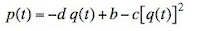(1)
where p(t) is the price of the commodity at time t and q(t) is the quantity supplied or demanded.

Time is discrete in this model, and the supply curve contains a lag. Firms plan the quantity to supply in the next period based on the price in this period:(2)
The supply curve shows "adaptive expectations". Economists such as Lucas have criticized the assumption of adaptive expectations. I think that critique may be inapplicable in a model with the behavior illustrated in Figure 5 below.

It's easy enough to solve for equilibrium, in which the quantity supplied and the quantity demanded are equal and do not change through time. Equation 3 gives the equilibrium quantity: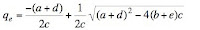(3)
Figure 1 illustrates. The supply and demand curves are shown. The solid dot is the equilibrium. A hint at the dynamics is also shown. At time t, the indicated quantity is thrown on the market. One reads the price at that time off the demand curve. The quantity supplied in the next period is found from drawing a horizontal line from that intersection with the demand curve to the supply curve. This point of intersection with the supply curve is the quantity supplied in the next period. Proceeding in this way, one draws a figure that resembles a cobweb. Thus, this model is known as the cobweb model.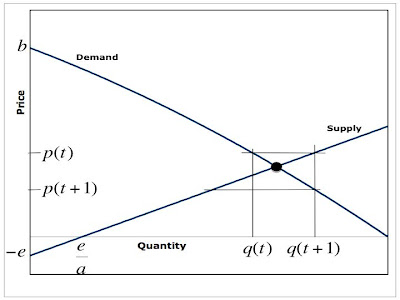Figure 1: Supply and Demand
I have explained the dynamics of this model above. One can approach the story with algebra and obtain a difference equation:(4)
Goodwin suggests redefining quantity as the deviation from the equilibrium quantity:(5)
where Q(t) is the redefined quantity. Equation 6 gives the difference equation in terms of the time path of the redefined quantity variable:(6)

3.0 Numerical Exploration of a Special Case

Goodwin considers the special case where the parameters b and e are both zero. Under this special case, the difference equation becomes considerably simplified: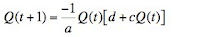(7)
Equation 7 resembles the logistic equation. As a start at exploring the dynamics of Equation 7, consider the case where a is unity, c is 3/5, and d is 21/20. (Update: Parameters were originally specified incorrectly.) Figure 2 shows time paths for two arbitrary initial values of the redefined quantity. Both time paths converge to a two-period limit cycle. The upper extreme of the blue time path continually falls, while the upper extreme of the red path continually rises. They meet in the limit at one point in the limit cycle. The lower extremes, shown in FIgure 2, converge to the other point in the limit cycle.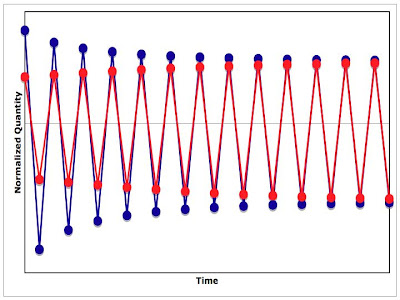Figure 2: Time Paths of Redefined Quantity
Figure 3 shows another method for illustrating these paths. The difference equation is graphed along with a 45-degree line through the origin. One starts at an initial point along the abscissa. Drop a line to the graph of the difference equation. The value of the ordinate at this intersection with the difference equation shows the value of the redefined quantity variable at the next instant in time. Draw a horizontal line from this intersection to the 45-degree line. The value of the abscissa at this intersection to the 45-degree line is, of course, the value of the redefined quantity variable at the next instant in time. Continually in this way, one can easily trace out a time path graphically. The limit cycle is drawn in Figure 3.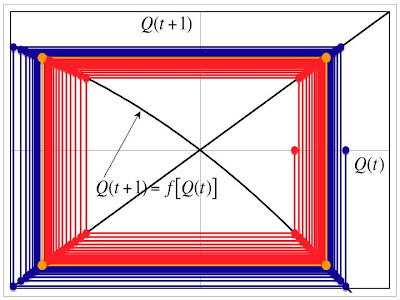Figure 3: Phase Space for These Paths
Structural dynamics concerns how the limiting behavior varies with the parameters of a difference or differential equation. Here I only consider the effects of a fall in a. Accordingly, Figure 4 shows the result when a is set to 7/10, with the other parameters as in Figure 3. The limit cycle has split into a cycle of period four. This period doubling sort of bifurcation is a common approach to chaos. For some lower values of a, the cycle will have periods eight, sixteen, thirty-two, etc. Figure 5 shows the result when a is 2/3. I believe Figure 5 is an example of chaos, where the limit is a non-wandering set with a fractal structure. (With more exploration, I would like to see a limit cycle of period three, or some other odd value. (Update: Try a as 0.57.) As I understand the mathematics, period doubling can only lead to such a limit cycle with intervening chaos.)Figure 4: Phase Space for Period Four Cycle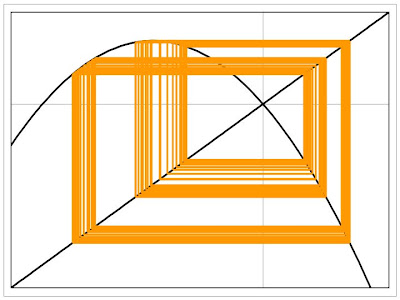Figure 5: Phase Space Showing Chaos

4.0 Conclusion

The above exposition begins with a common introductory model in economists. And it ends with mathematical chaos. Chaos is shown in a special case in which both the demand and the supply curves go through the origin. A supply curve going through the origin, although a special case, is quite reasonable in economics. It is not economically sensible for the demand curve to go through the origin.

If there were to be a part 2 for this post, it would demonstrate the possibility of chaos in an economically relevant parameter range. One would want the demand curve to be declining throughout the first quadrant as well as intersecting the price axis at a strictly positive price. And one would want the strange attractor to lie entirely in the first quadrant for the price and untransformed quantity variables. But I haven't done enough numerical exploration yet.

Gabriel M said...

Huh... could you please post the equations for supply, demand and the law of motion you used? (I don't have access to Goodwin's book.)

Even with a Ricatti, reduced-form law of motion for price, you shouldn't get anything funky for relevant parameter ranges, as far as I can remember.

The reference for the critique here is not Lucas, I think, but rather Muth's original RE article, which was, after all, on Kaldor's hog cycle, IIRC--in any case, something very much related.

For producers to decide how much to supply by taking only today's price into account... those are pretty naive expectations :-) They should be able to form a better forecast even if you don't go the RE route.

YouNotSneaky! said...

Gabriel's right, at least about the first part. Roughly, to get more than one-period cycle with parameters that make sense, you'd need the q_t+1(q_t) equation to be concavely non-monotonic in the first quadrant. Basically you'd need something like
q_t+1 = a + b*q_t - c*q_t^2
with a,b, c >0. This of course comes from the price equations:
p_t = m1 - m2*q_t - m3*q_t^2 and
p_t = n1 + n2*q_t+1
with the "relevant parameters" here all being non negative except for n1 which can go either way. So solving for q_t+1 as function q_t above we get:
a = (m1-n1)/n2 > 0 (even if n1>0 as long as demand has higher intercept than supply)
b = - (m2/n2) < 0
c = (m3/n2) > 0

a and c work but not b - you can't get non-monotonicity. So for "relevant parameters" you can get cobweb, one period cycle and "world falls apart" but not multi-period cycle. And since chaos (I hope I don't get slapped by some math person for saying this) is essentially a "infinite-period" cycle, I don't think you can get it in this formulation.

You could get it if you do some stretching and assume you're talking about some funky good such that m2<0 (so that for small quantities demand initially increases with price - maybe that's why things like fashion are so unstable). More realistically you can probably get chaos in a multi-good general equilibrium model where the shape of the difference function will depend on cross elasticities of substitution.

If you got a stable cycle then "adaptive expectations" don't really make sense since the same thing keeps happening over and over. Whether they make sense in the presence of chaos is a religious question though.

Of course my math could be wack. It's been happening a lot lately for some reason.

Robert Vienneau said...

Equation 3 is in error. Both terms in the square root should be positive. Equation 6 is correct.

Robert Vienneau said...

Consider the case, in the notation of my post, where a = 1, b = 781/960, c = 3/5, d = 1/2, and e = 0. This yields of stable and attracting period 2 cycle in the first quadrant. Cleary younosneaky's math is wacked.

I don't know whether one can get the whole period-doubling scenario leading to chaos in the first quadrant. If not, one might want to investigate higher-order polynomials for supply and demand that are still monotonic in the appropriate region (from the origin to the first intersection of the demand curve with the quantity axis) in the first quadrant.

Gabriel M said...

Ooops... Now I see that you already posted everything I asked. Sorry.

I can't find the time to look at this seriously until the weekend. :-(

YouNotSneaky! said...

Weird, previous comment didn't show up.
Anyway. I don't think you get a cycle in this one. Rather it unravels and q_t -> 0 as t-> inf. It's still monotonic in first orthant so no cycles.

Robert Vienneau said...

I don't think anybody is obligated to look seriously at anything I post.